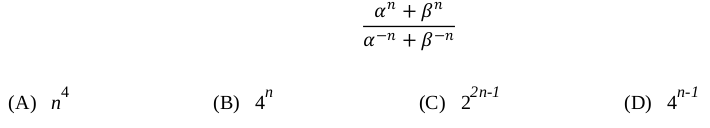Open in App
Not now

# GATE | GATE-CS-2016 (Set 2) | Question 5

• Last Updated : 28 Jun, 2021

In a quadratic function, the value of the product of the roots (α, β) is 4. Find the value of(A) A
(B) B
(C) C
(D) D

Explanation:
(α^n + β^n)/ (1/α^n +1/β^n)

(α^n + β^n)/ (β^n + α^n )*(α^n * β^n)

(α^n * β^n)

αβ^n

4^n

This explanation has been contributed by Dharmesh Singh.

Quiz of this Question

My Personal Notes arrow_drop_up
Related Articles